# 电源方案（Buck）- LMR14050

LMR14050 是 TI 的一款 Buck 转换器芯片，输入电压范围很宽（4-40V），且能提供 5A 的持续输出电流，轻载有休眠模式提高效率。它的内部集成度高，所以外围需要设计的元器件很少。开关频率能通过外部电阻 $$R_T$$ 在 200kHz-2.5MHz 范围内选择，也能够与 250 kHz-2.3 MHz 频率范围内的外部时钟同步。保护功能有过温关断、$$V_{OUT}$$ 过压保护（OVP）、$$V_{IN}$$ 欠压锁定（UVLO）、逐周期电流限制和带频率折返的短路保护。

## 主要特性

• 拓扑：DC/DC（Buck）
• 器件型号：LMR14050SDDA
• 封装：HSOIC-8
• 输入电压：4-40 V
• 输出电压：0.8-28V
• 输出电流： 5A 持续
• 工作频率： 200kHz-2.5MHz
• 参考价格：￥ 11.3
• 其他特性
• 40µA 超低工作静态电流
• 关断电流：1µA
• 90mΩ 高侧 MOS 管
• 最短导通时间：75ns
• 电流模式控制
• 热保护、过压保护和短路保护

## 内部功能框图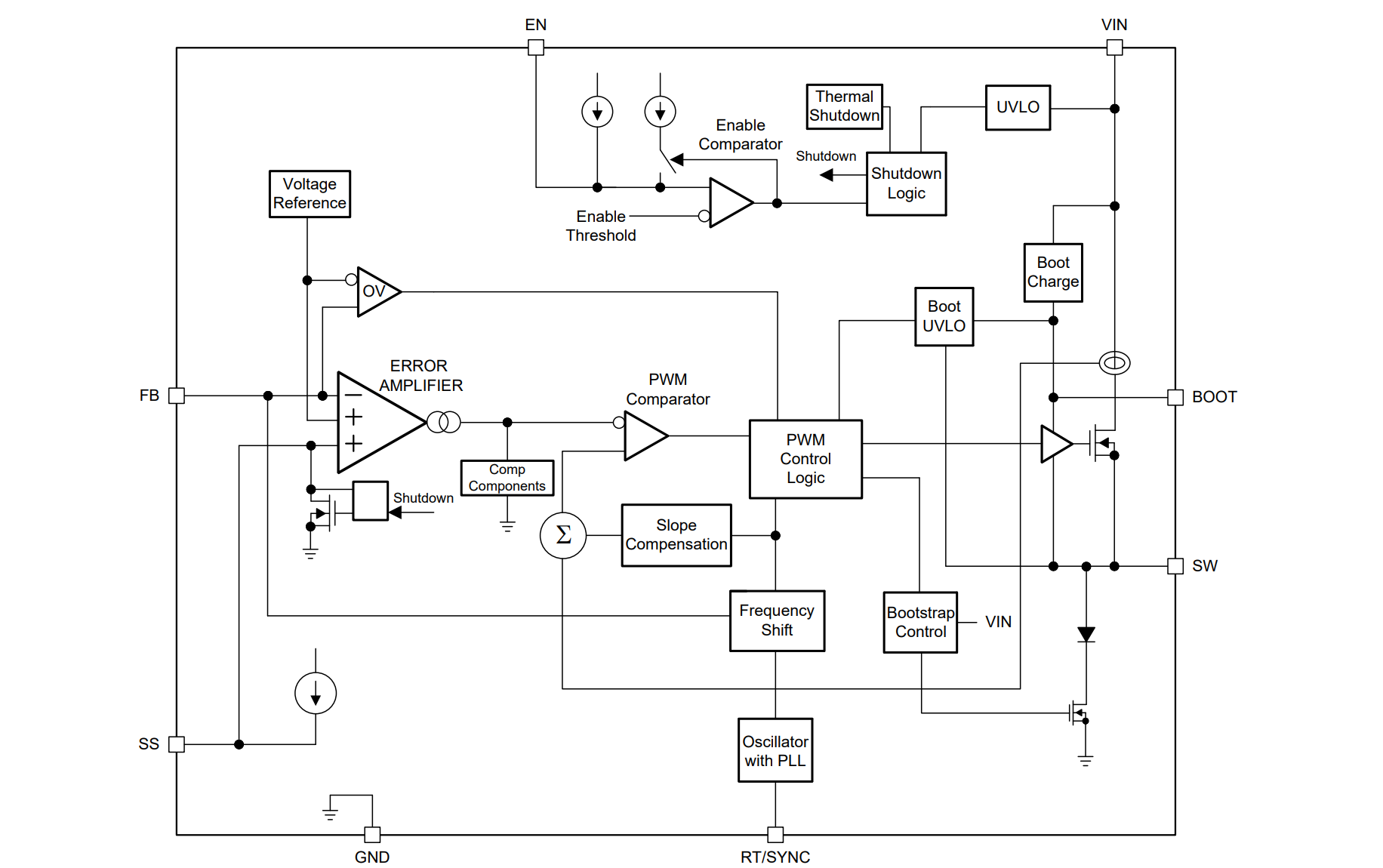## 引脚定义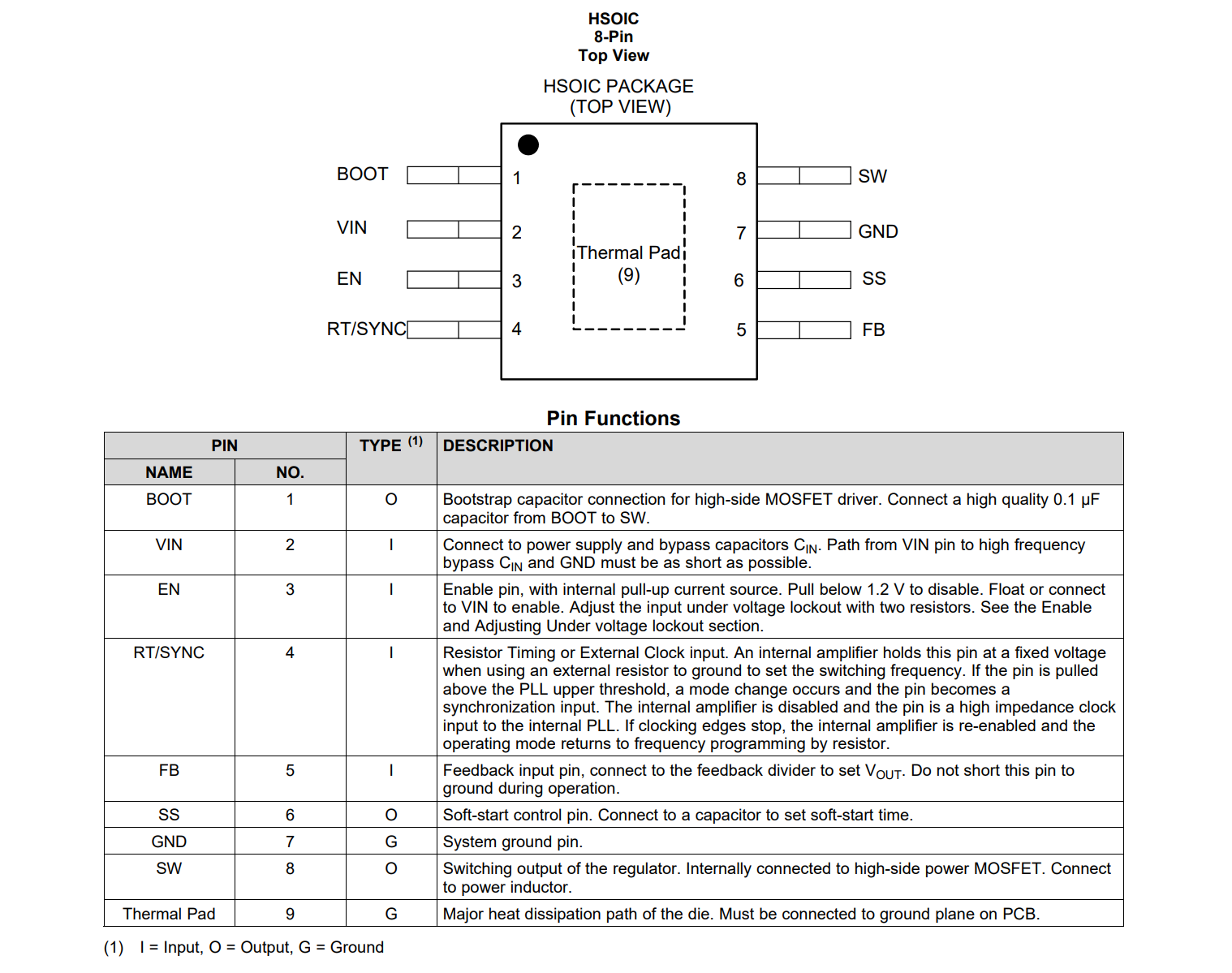• BOOT：给高侧 MOS 管的自举电容。在 BOOT 和 SW 间接一个 0.1uF 电容。
• VIN：电源输入，经过去耦电容 $$C_{IN}$$ 后接到此引脚。
• EN：使能开关，内部上拉。外部拉低于 1.2V 可关闭输出，浮空或接 $$V_{IN}$$ 使能输出。欠压锁定的调节请见下文。
• RT/SYNC：电阻时序或外部时钟输入。当使用外部电阻接地来设置开关频率时，内部放大器将此引脚保持在固定电压。如果引脚被拉至高于 PLL 上限阈值，则会发生模式更改并且引脚变为同步输入。内部放大器被禁用，引脚是内部 PLL 的高阻抗时钟输入。如果时钟边沿停止，则重新启用内部放大器并且操作模式返回到通过电阻器进行的频率编程。？
• FB：反馈输入引脚，由电阻从 $$V_{OUT}$$ 分压输入反馈，不可直接接地。
• SS：缓启动控制引脚，接电容设置缓启动时间。
• SW：稳压开关输出，在内部连高侧 MOS 管。接功率电感。

## 特性描述

### 稳压原理

LMR14050 的输出电压通过开启高侧 N-MOS 并控制导通时间来调节。在高侧 N-MOS 导通期间，SW 引脚电压摆动至大约 $$V_{IN}$$，电感电流 iL 随线性斜率 ($$V_{IN}$$$$V_{OUT}$$) / L 增加；当高侧 N-MOS 关断时，电感电流通过续流二极管，以 $$V_{OUT}$$ / L 的斜率放电。稳压器的控制参数由占空比 $$D = t_{ON} /T_{SW}$$ 决定，其中 $$t_{ON}$$ 是高端开关导通时间，TSW 是开关周期。稳压器控制环路通过调整占空比 D 来保持恒定的输出电压。在理想的降压转换器中，损耗被忽略，D 与输出电压成正比，与输入电压成反比：$$D = V_{OUT} / V_{IN}$$### BOOT 自举电路的设计

LMR14050 内部集成了自举电压转换器，在 BOOT 和 SW 引脚接一个自举电容，就可以提供足以驱动高侧 MOS 管门极的电压。BOOT 电容的参考值为 0.1uF（X7R 或 X5R 陶瓷电容，耐压至少 16V）。

### 输出电压调节

LMR14050 提供一个 0.75V 的内部参考电压。输出电压通过电阻分压器，从 $$V_{OUT}$$ 分压出来输入 FB 引脚，在内部进行比较调节。分压电阻建议使用偏差 1% 或更低的、温度系数 100 ppm 或更低的。通过所需分压电流选择低侧电阻 $$R_{FBB}$$（参考值是 10-100kΩ），并通过公式计算高侧电阻 $$R_{FBT}$$。选择较大的阻值有利于提高轻载效率，但如果太大，稳压器将更容易受到来自 FB 输入电流的噪声和电压误差的影响。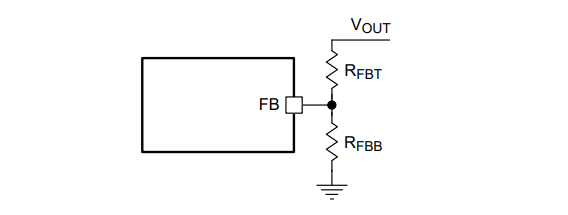$R_{FBT}=\frac{V_{OUT}-0.75}{0.75}R_{FBB}$

### EN 使能与欠压锁定调节

$$V_{IN}$$ 高于 3.7V，且 EN 高于 1.2V 阈值时 LMR14050 开启输出，当 $$V_{IN}$$ 掉落到低于 3.52V 或 EN 低于 1.2V 时稳压器关闭。EN 有内部上拉电流源（1uA）以确保 EN 脚浮空时稳压器正常输出。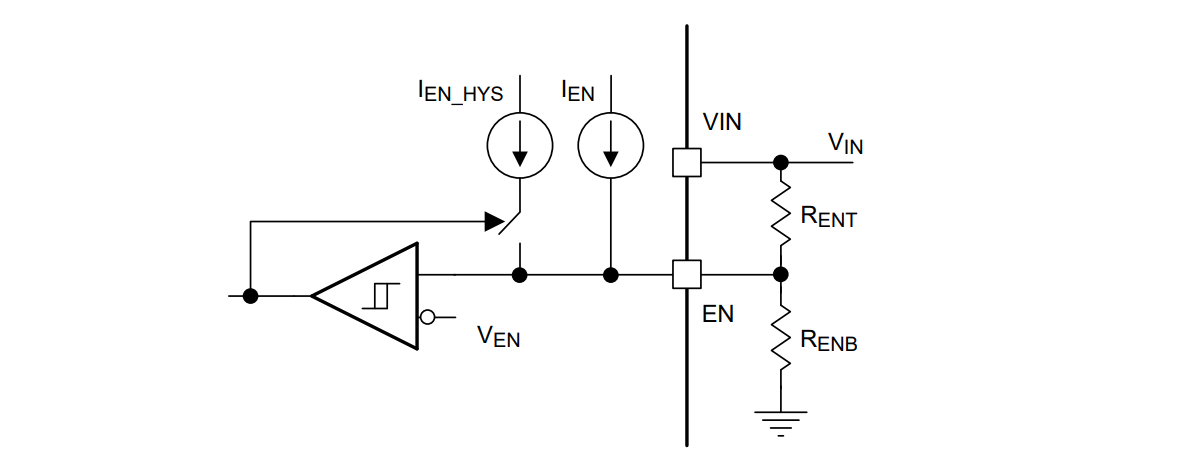$$R_{ENT}$$$$R_{ENB}$$ 遵从以下公式计算：

$R_{ENT}=\frac{V_{STRAT}-V_{STOP}}{I_{HYS}}$

$$R_{ENB}=\frac{V_{EN}}{\frac{V_{START}-V_{EN}}{R_{ENT}}+I_{EN}}$$

### 外部缓启动

$$t_{SS}(ms)=\frac{C_{SS}(nF)*V_{REF}(V)}{I_{SS}(uA)}$$

### 开关频率与同步（RT/SYNC）

LMR14050 的开关频率可以被接在 RT/SYNC 和 GND 之间的电阻 $$R_T$$ 编程设定。RT/SYNC 引脚不可浮空或端接到地，根据以下公式或图表决定其阻值：

$R_T(kΩ)=32537*f_{SW}^{-1.045}(kHz)$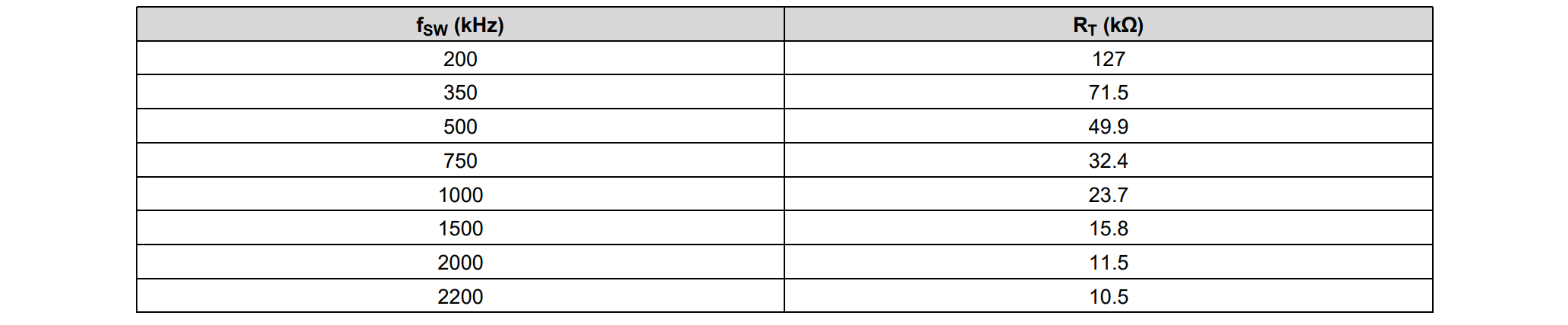LMR14050 开关动作也可以被外部时钟输入信号同步（250kHz-2.3MHz）: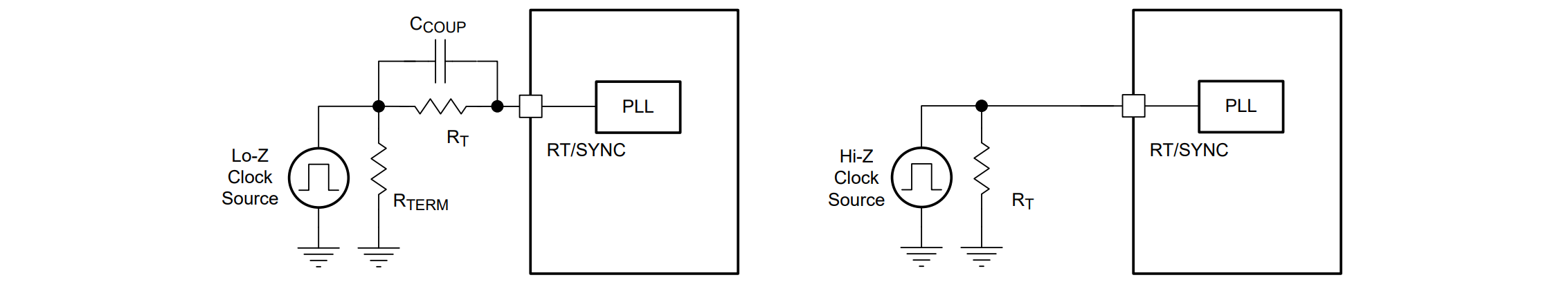### 过流与短路保护

LMR14050 通过对高侧 MOS 管的峰值电流进行逐周期电流限制，以防止过流情况。每个开关周期都会将高端开关电流与误差放大器（EA）的输出减去斜率补偿进行比较。高侧开关的峰值电流受限于恒定的钳位最大峰值电流阈值。因此，高侧开关的峰值电流限制不受斜率补偿的影响，并且在整个占空比范围内保持恒定。

### 过压保护

LMR14050 内置输出过压保护（OVP）电路，以最大限度减少电压过冲。当 FB 电压达到上升 OVP 阈值（VREF 的 109%）时会关闭高侧 MOS 管；当降至低于 OVP 下降阈值（VREF 的 107%）时，高侧 MOS 管恢复正常工作。

### 热关断保护

LMR14050 有内部热关断保护功能。当结温超过 170℃ 时热关断激活，高侧 MOS 管停止开关。当芯片温度降至 158℃ 以下，才会从内部软重启。

## 参考设计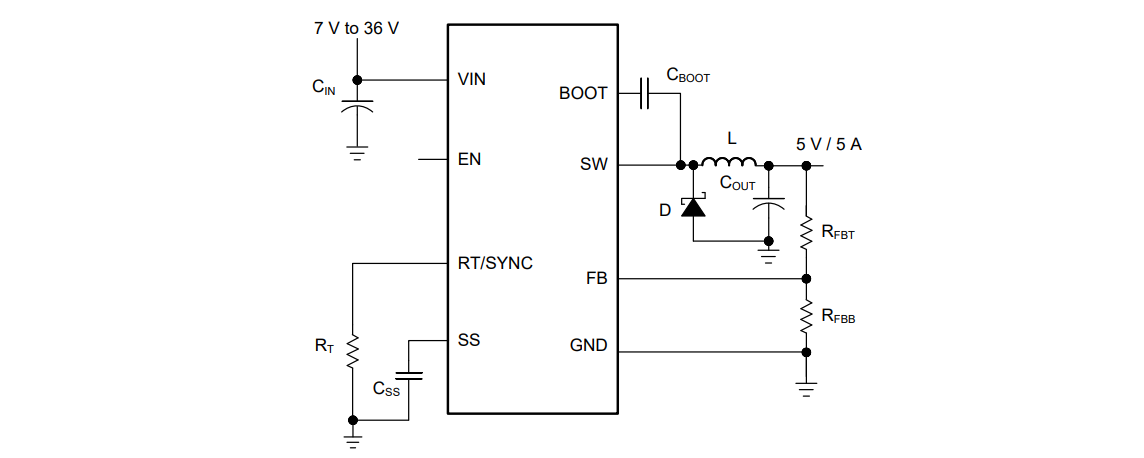• 输入电压 $$V_{IN}$$：7-36V，典型值为 12V
• 输出电压 $$V_{OUT}$$：5V
• 最大输出电流 $$I_{O\_MAX}$$：5A
• 瞬态响应（0.5-5A）：5%
• 输出电压纹波：50mV
• 输入电压纹波：400mV
• 开关频率 $$f_{SW}$$：300kHz
• 缓启动时间：5ms

### 输出电感选型

$Δi_L=\frac{V_{OUT}*(V_{IN\_MAX}-V_{OUT})}{V_{IN\_MAX}*L*f_{SW}}$
$L_{MIN}=\frac{V_{IN\_MAX}-V_{OUT}}{I_{OUT}*K_{IND}}*\frac{V_{OUT}}{V_{IN\_MAX}*f_{SW}}$

### 输出电容选型

$ΔV_{OUT\_ESR}=Δi_L*ESR=K_{IND}*I_{OUT}*ESR$

$ΔV_{OUT\_C}=\frac{Δi_L}{8*f_{SW}*C_{OUT}}=\frac{K_{IND}*I_{OUT}}{8*f_{SW}*C_{OUT}}$

$$C_{OUT}>\frac{3*(I_{OH}-I_{OL})}{f_{SW}*V_{US}}$$

$$C_{OUT}>\frac{I_{OH}^2-I_{OL}^2}{(V_{OUT}+V_{OS})^2-V_{OUT}^2}*L$$

• $$K_{IND}$$ 为电感纹波电流的纹波比（$$Δi_L/I_{OUT}$$
• $$I_{OL}$$ 为负载瞬态期间的低电平输出电流
• $$I_{OH}$$ 为负载瞬态期间的高电平输出电流
• $$V_{US}$$ 为目标输出电压下冲
• $$V_{OS}$$ 为目标输出电压过冲

### 输入电容选型

LMR14050 需要高频输入去耦电容和大容量输入电容。高频去耦电容的典型推荐值为 4.7-10 μF（X5R/X7R，陶瓷电容，耐压为最大输入电压两倍以上）。在参考设计中，使用了两个额定电压为 100 V 的 2.2 μF X7R 陶瓷电容器。高频滤波电容需要靠近稳压器放置。

### BOOT 自举电容选型

LMR14050 需要一个 BOOT 自举电容，在前文有提及，BOOT 电容的参考值为 0.1uF（X7R 或 X5R 陶瓷电容，耐压至少 16V）。

## Layout 参考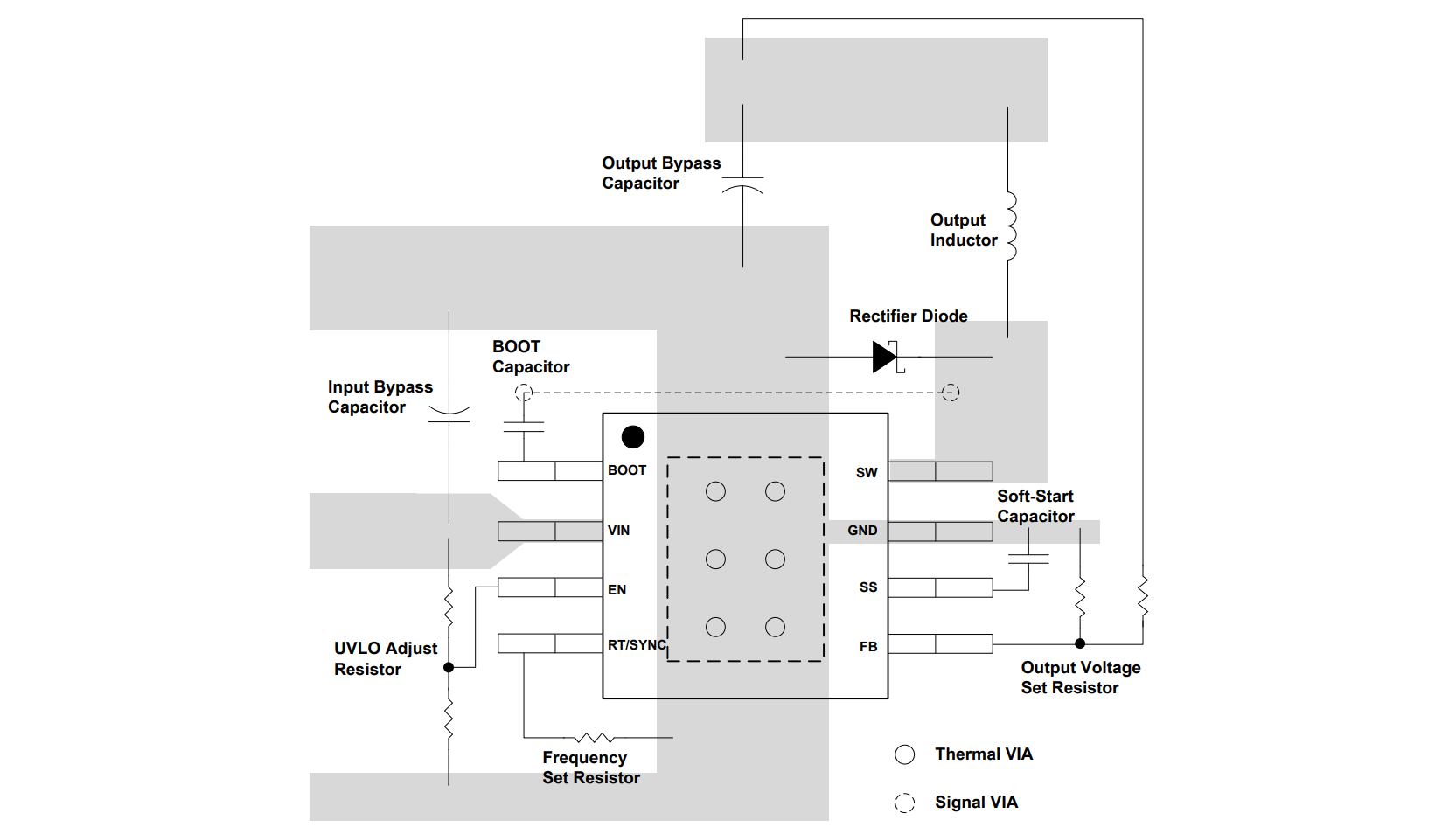1. 反馈网络、电阻 $$R_{FBT}$$$$R_{FBB}$$ 应尽量靠近 FB 引脚。 $$V_{OUT}$$ 的采样路径应远离噪声产生路径，最好通过屏蔽层另一侧的层。
2. 输入去耦电容需要尽可能靠近 $$V_{IN}$$ 和 GND 放置。
3. 电感应靠近 SW 引脚放置，以减少磁噪声和静电噪声。
4. 输出电容 $$C_{OUT}$$ 应靠近电感和二极管的节点放置，走线尽可能短，以降低传导和辐射噪声，提高效率。
5. 二极管、$$C_{IN}$$$$C_{OUT}$$ 的接地连接应尽可能小，并仅在一个点（最好在 $$C_{OUT}$$ 接地点）连接到系统接地层，以最大限度地减少系统接地层中的传导噪声。

## 实际测试

24V 输入，5V/5A 满载输出，实际输出 4.95V/5.00A，纹波 15mV，温度 110℃。